# Polarization Index Test and DAR Test

Polarization index test is 10 minutes to 1 minutes ratio test of insulation resistance. Similarly, DAR test is 60 seconds to 30 seconds insulation resistance ratio test. The advantage of insulation resistance time ratio test is cancellation of measurement error caused by temperature. Therefore, PI test and other ration test DAR test are most accurate.Polarization Index Test or PI test is conducted on high voltage electrical equipment to ensure the insulation quality. The polarization index test shows about the dryness and cleanliness of insulating surface.

Conductors are used for transmitting the electrical energy from the generating end to utilization end. However, without use of insulating materials, it is impossible to transmit and utilize the electrical energy. The quality of the insulating material is a key factor for reliable operation of electrical equipment. About 98 % faults occur on account of insulation failures and single phase to earth fault has

Most of the insulating materials are hygroscopic in nature and absorbs the atmospheric moisture present in the air. The absorbed moisture can dehydrates with rise in temperature of winding of electrical machines, and as a result insulator may become brittle. Furthermore, the resistance of the insulating material gets deteriorated with rise in temperature.

The useful life of the insulating material gets halved with every 10 degree centigrade rise in temperature. The temperature of insulating material gets increased with higher loading on current carrying conductor, with higher loading on electrical machines, or with improper ventilation, where the equipment are installed.

As the operational reliability of electrical equipment like motor, generators mainly depends on the insulation, the health of the insulation is required to be periodically checked and ascertained. Insulation Resistance (IR) and Polarization Index (PI) tests are two methods widely used for ensuring the healthiness of insulation system.

Higher value of insulation resistance indicates that there is negligible leakage current through the insulator. The lower IR indicates that the insulation has deteriorated. The IR measurement is just indicative of the insulating quality. When the DC voltage (V) is applied across the insulator the current (I) flows through it.

Insulation Resistance (IR)

R = V/I

Where, R = Insulation Resistance

V = Voltage applied

I = Current passing through insulating material

The measurement value of insulation resistance depends on the period of time for which voltage is applied because the current drawn by the insulating material decays with time. When the IR is taken initially and after one minute, the initial IR value will be different than the IR value taken after one minute.

The reason of this is that the insulator takes high current initially when the voltage is applied across it, and the current decays exponentially with time. The initial IR value will be less than the value of IR after one minute. Therefore, IR value should be taken after applying the voltage for one minute for accurate measurement of IR. The insulation resistance test does not give the correct reading and some advanced tests are carried out to ascertain the quality of insulating materials and remaining useful life of the insulation.

The  following advanced tests are performed to ensure the quality of insulation.

1. Polarization Index Test
2. Dielectric Absorption Ratio (DAR) or DA Test

## What is a Polarization Index or PI?

To ascertain the quality of insulation one very important data known as polarization index is required to be checked. Polarization Index is the ratio of  insulation resistance after 10 minutes and 01 minute.The Polarization Index formula is as given below.

## Polarization Index Formula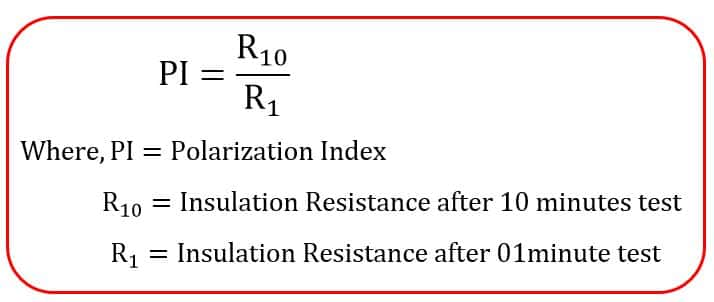### How to perform polarization Index (PI) Test?

For determining PI, the insulation resistance is measured at different times after starting of the test. The DC voltage is applied between the conductor and insulation, then the insulation resistance is measured after 01 minute and 10 minutes of starting of the test. If  insulation resistance is 300 MΩ and 600 MΩ after 01 minute and 10 minute respectively. The PI is 600/300 = 2

### Mechanism of Polarization Current in Insulating Material

When voltage is applied across insulator the flow of current in the insulator can be broadly categorized into four categories. There are four components of current decides the polarization index of insulating materials.

### Capacitive Current (IC)

Insulator acts as a capacitor. When the DC voltage is applied to capacitor, it draws high charging current and when the all the dielectric gets polarized the current reduced to zero. The charging current decays at exponential rate. In case of generator or motor winding, the charging current decays at faster rates and it effectively decays to zero in less than 10 seconds. The IR value must be taken after decay of capacitive charging current for correct measurement of insulation resistance.

### Conduction Current( IR )

This current is due to the flow of electrons between the conducting material and the insulating material. This is galvanic current through ground wall. Such a current can flow if the ground wall has absorbed moisture, which can happen on the older thermoplastic insulation systems.

The current may also flow if there are cracks, cuts or pinholes in the ground insulation and some contamination is present to allow current to flow. This current is constant with time. With modern insulation this current usually is zero (as long as there no damage to the insulation).

### Surface leakage Current(IS)

This is constant DC current that flows over the surface of the insulation. It is caused by conductive contamination (oil or moisture mixed with dust, dirt, insects, chemicals etc) on the surface of the winding. This current is also constant with time.

### Polarization Current(IP)

Electrical insulation is hygroscopic in nature and presence of moisture will be there either in low quantity or in excess. When an electric field is applied across the insulation, it starts absorbing electrons from the hydrogen molecules causing ionization of hydrogen. The molecules on the insulator containing water start aligning in the electric field.

The energy required to align the molecules comes from the current in the DC test voltage supply. This current is called polarization current. The water becomes completely polarized when the absorption of electron from hydrogen merging with oxygen is completed. Once the molecules get aligned, the current stops. The approximate time for complete polarization is 10 minutes. That is why the IR is measured after 10 minutes of applying voltage.

### Significance of Polarization Index Test

From above discussion, it is clear that insulator must be fully polarized and the leakage current and conduction current should reduce.

Insulation resistance after 01 minute IR test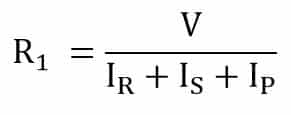Insulation resistance after 01 minute IR test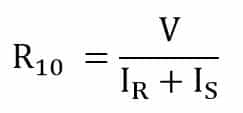The polarization index is;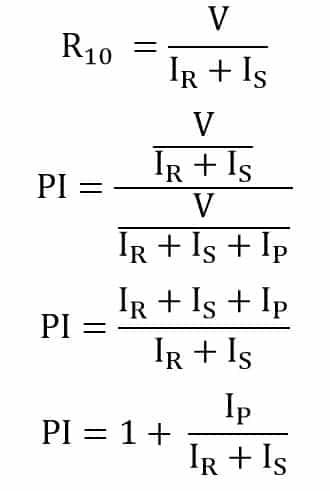If the value of (IR + IS ) is low compared to polarization current(IP), then PI value is more than 1. The value of  (IR + IS )<< IP for better PI.

### A low value of PI indicates that the winding is contaminated with oil, dirt or winding have absorbed moisture. The polarization Index curve is as shown below.

Example:

#### Polarization Index calculation of a generator stator winding

 S.No DETAILS VALUE(GΩ) 1 Test Type IR & PI 2 Test 5000 Volt Phases R-(Y+B+E) Y-(B+R+E) B-(R+Y+E) 3 15” 3.88 3.81 3.68 30” 6.23 6.82 6.42 45” 7.86 8.1 8.23 1.00” 9.84 9.97 10.1 2.00” 14.7 15.2 14.8 3.00” 18.8 19.5 18.2 4.00” 22.1 20.8 19.6 5.00” 22.5 22.1 21.8 6.00” 23.2 23.6 24.2 7.00” 26.5 24.3 26.5 8.00” 28.7 26.5 27.9 9.00” 29.8 28.3 31.7 10.00” 33.6 29.6 33.9 4 PI Value 3.38 2.96 3.36
What should be the Minimum PI (Polarization Index) Value?

The Polarization Index acceptable values of the insulation is given in below table.This is the general guideline for interpreting the polarization index test results. The PI value should be more than 2.
 Interpretation of Polarization Index results PI Condition of item under test 1 Hazardous 1 – 1.5 Bad 1.5 – 2.0 Doubtful 2.0 – 3.0 Adequate 3.0- 4.0 Good 4 and above Excellent

## Dielectric Absorption Ratio (DA or DAR) Test

DAR or DA test  is also the ratio test carried out to determine the quality of the insulation. The DAR test is conducted for lesser time than the PI test. The instantaneous insulation resistance test does not give the exact reading because the insulating material draw charging current initially and after some time it decays. The capacitive current must decay within 01 minute if insulation is healthy.

## Dielectric Absorption Ratio Formula

Dielectric Absorption Ratio (DA or DAR)  is the ratio of insulation resistance after 60 seconds and 30 seconds.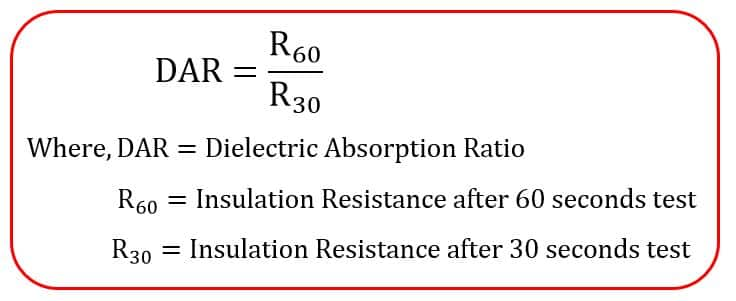### How to perform DAR Test?

For determining DAR or DA, the insulation resistance is measured at different times after starting of the test. The DC voltage is applied between the conductor and insulation, then the insulation resistance is measured after 30 seconds and 60 seconds of starting of the test. If  insulation resistance is 100 MΩ and 25 MΩ after 60 seconds and 30 seconds respectively.
The DAR is 100/25 = 4

## Advantage of PI and DA Test

The insulation resistance of the insulating material decreases with increase of temperature. Thus, the spontaneous value of IR depends on the temperature of the insulating material. That is why the one reading of IR value does not give the accurate result.
The PI and DA test is the ratio test and temperature remain the same in both the readings taken at different time, and the measurement is independent of temperature. Therefore, PI and DA test gives accurate result.